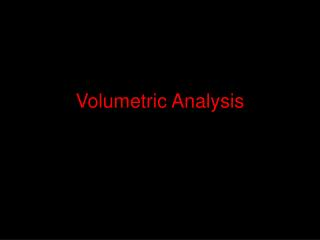DownloadDownload PresentationVolumetric Analysis

Volumetric Analysis

Télécharger la présentationVolumetric Analysis

- - - - - - - - - - - - - - - - - - - - - - - - - - - E N D - - - - - - - - - - - - - - - - - - - - - - - - - - -
Presentation Transcript

1. Volumetric Analysis

2. Volumetric Analysis • Volumetric analysis is the method of finding out the concentration of a solution. • For example: • Adding a solution of acid to a solution of base, in a measured way, until you have added enough to neutralise the base (which an indicator will tell you) • Using an indicator like this to find exact amounts of solutions that react is called is called titration.

3. Titration • In this method, the concentration of one of the two solutions must be known – this we call the standard solution (and it never changes with time). • Knowing the concentration of one of the solutions, and the exact volumes of both, will allow you to work out the unknown concentration. • E.g. looking back at the earlier example: if we know the volume of acid needed to neutralise the base; and the volume of the base the acid is being added to and the concentration of the base; then we have all the info. we need to work out the acid concentration. • The base solution is the standard solution because we know its volume and its concentration.

4. Titration Method • Usually 25.0 cm3 of the standard solution is known. So for example, a pipette would be used to deliver this amount of the base (NaOH) into a clean conical flask. • Add a few drops of indicator (e.g. phenolphthalein) • Wash burette with a little bit of acid. Then fill the burette with acid, until meniscus is at zero. • Run the acid into the conical flask, until indicator changes colour to show neutralisation has been reached – call this the end-point.

5. Titration Method • First run is normally a rough one to see where end point will roughly be on burette. After this the titration is repeated more accurately, with acid being run into base quickly up to the near the end-point, and then drop by drop to find the exact volume of acid that causes neutralisation. • The ‘titre’ is the volume of acid needed to neutralise 25cm3 of the base. • Use the average titre obtained from the repeat runs in calculation. First run ignored whhen working out average.

6. Key Notes

7. Calculation Example: • By titration you find that 15.0 cm3 of hydrochloric acid neutralise 25.0 cm3 of a 0.100 mol dm-3 solution of sodium hydroxide. What is the concentration of hydrochloric acid? • First you always need equation for reaction first!!!: • Hydrochloric acid + sodium hydroxide → sodium chloride + water • HCl(aq) + NaOH(aq) → NaCl(aq) + H2O(l) • This tell you that 1 mole of HCl neutralises 1 mole of NaOH. • Next work out the moles for either the base or the acid. • You know the concentration for the base, so you start there

8. Amount (mol) = volume (dm3) x concentration (mol dm-3) • Amount (mol) NaOH = 25.0 cm3 x 0.100 mol dm-3 = 2.50 x 10-3 mol. • Now work out concentration of acid: • From equation; amount (mol) of HCl = amound (mol) of NaOH = 2.50 x 10-3 mol. • Amount (mol) = volume (dm3) x concentration (mol dm-3) • Re-arrange equation to give: • Concentration HCl = amount (mol) / volume (dm3) • Concentration HCl = 2.50 x 10-3 mol / 15.0 cm3 • Concentration HCl = 0.167 mol dm-3.

9. Another Example Volume of 250 cm3 of acid was 3.02g Answer next slide

10. REDOX titrations • A titration, where the reaction is not a neutralisation, but a redox reaction. • Major example is reaction between iodine and thiosulphate ions. • Reaction is carried out the same way as a acid-base titration.

11. REDOX titrations

12. Finding the purity of potassium iodate(V) The iodine can be generated by adding iodate(V) ions to excess potassium iodide solution. Iodate(V), IO3-, reacts as a strong oxidising agent. This mixture above can be titrated with standard sodium thiosulphate solution. STARCH is the indicator! (blue or blue-black to colourless)

13. So 0.001 mol of I2 liberated by 25cm3 of impure potassium iodate(V) solution Mol = 0.000333 mol 0.001 mol V = 25 cm3 V = 20 cm3 M = 0.1 mol dm-3 Start here 0.001 mol Mol = 0.002 mol

14. Mol = 0.000333 mol 0.001 mol V = 25 cm3 V = 20 cm3 M = 0.1 mol dm-3 0.001 mol Mol = 0.002 mol

15. Finding the Percentage of copper in brass • Redox titration can be used in the analysis of brass (an alloy of copper and zinc)

16. Questions